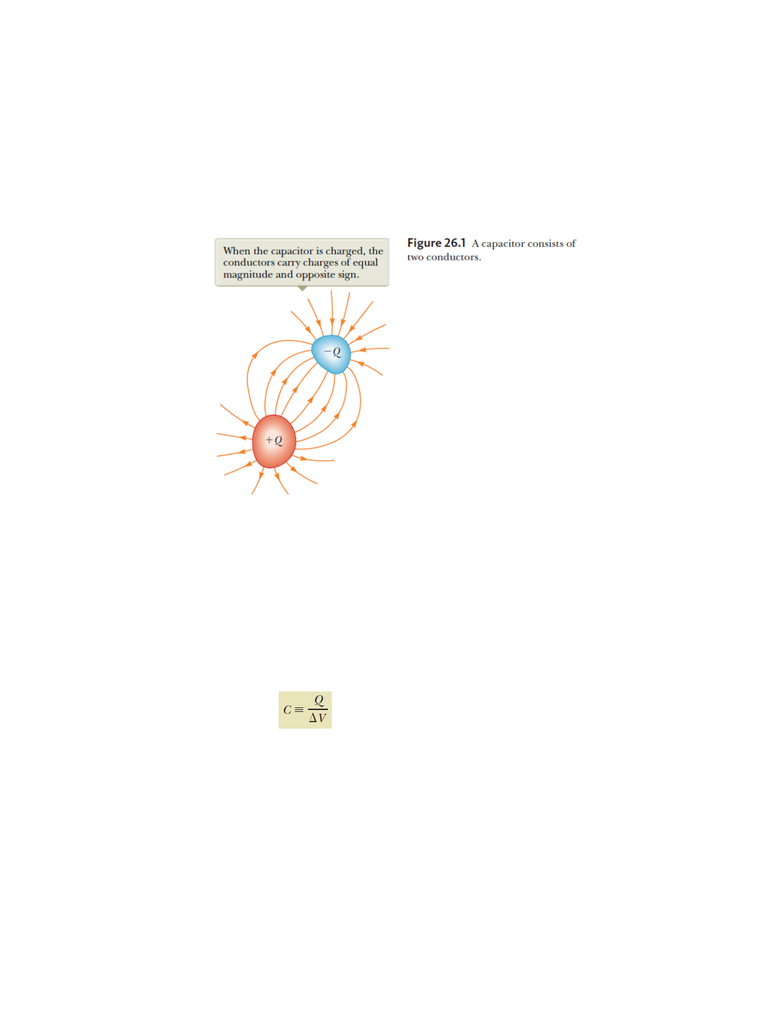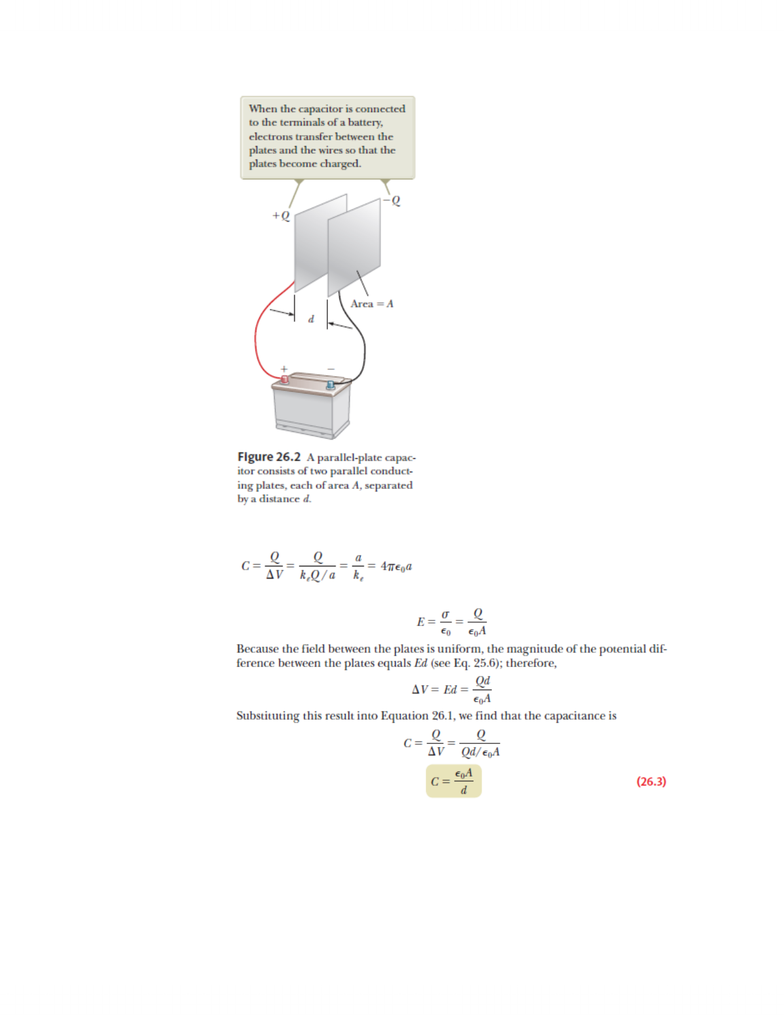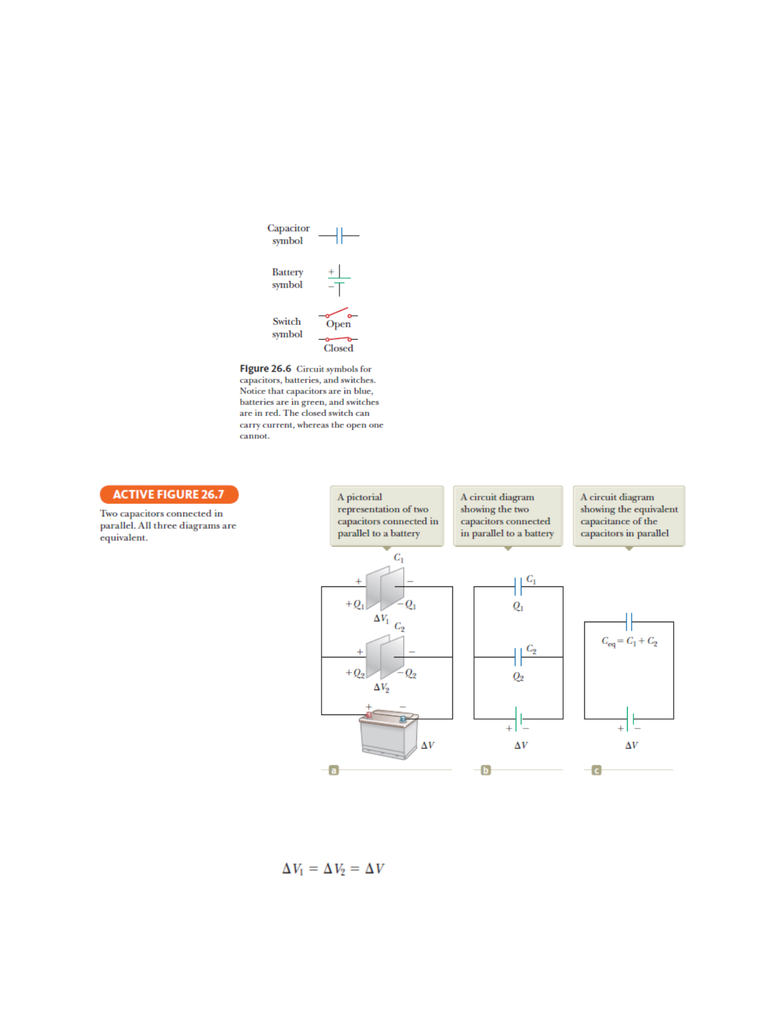Textbook Notes (280,000)
CA (170,000)
McGill (5,000)
Physics (60)
Chapter 26

# PHYS 142 Chapter Notes - Chapter 26: Wax Paper, Electrolytic Capacitor, Dielectric

Department
Physics
Course Code
PHYS 142
Professor
Yoichi Miyahara
Chapter
26

This preview shows pages 1-3. to view the full 9 pages of the document.1
PHYS 142 Chapter 26 Smart Notes
26.1: Definition of Capacitance
o Capacitors
A combination of two conductors is called a capacitor. The conductors are called
plates. If the conductors carry charges of equal magnitude and opposite sign, a
potential difference exists between them.
o Capacitance
Definition
The capacitance C of a capacitor is defined as the ratio of the magnitude
of the charge on either conductor to the magnitude of the potential
difference between the conductors
To understand capacitance, think of similar notions that use a similar
word. The capacitance of a capacitor is the amount of charge the
capacitor can store per unit of potential difference.
Capacitance is always a positive quantity.
Equation
SI Units

Only pages 1-3 are available for preview. Some parts have been intentionally blurred.2
26.2: Calculating Capacitance
o Capacitance of an Isolated Charged Sphere
o Parallel Plate Capacitors
As a capacitor is being charged by a battery, electrons flow into the negative
plate and out of the positive plate.
Consider the situation before any charges have had a chance to move in
response to this change. Because no charges have moved, the electric field
between the plates has the same value but extends over a shorter distance.

Only pages 1-3 are available for preview. Some parts have been intentionally blurred.3
Therefore the magnitude of the potential difference between the plates is
smaller.
When the potential difference between the plates again matches that of the
battery, the flow of charge stops.
26.3: Combinations of Capacitors
o In studying electric circuits, we use a simplified pictorial representation called a circuit
diagram. Such a diagram uses circuit symbols to represent various circuit elements.
o Parallel Combination of Capacitors
The individual potential differences across capacitors connected in parallel are
the same and are equal to the potential difference applied across the
combination.
Sum of the charges on the individual capacitors